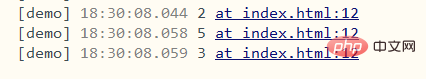# javascript中求根的函数有哪些

javascript中求根的函数：1、sqrt()函数，语法“Math.sqrt(x)”，可计算数x的平方根；2、pow()函数，语法“Math.pow(x,(1/y))”，可计算数x的y次根。javascript求根

1、使用sqrt()函数

sqrt() 方法返回数的平方根。

`Math.sqrt(x)`

`var x=9; console.log(Math.sqrt(x));`2、使用pow()函数

`Math.pow(x,y)`可 x 的 y 次幂。当y为小数（1/y）时，可用于开根号计算。

`function f(x,y){ 	y=1/y; 	console.log(Math.pow(x,y)); } f(4,2); f(125,3); f(81,4);`